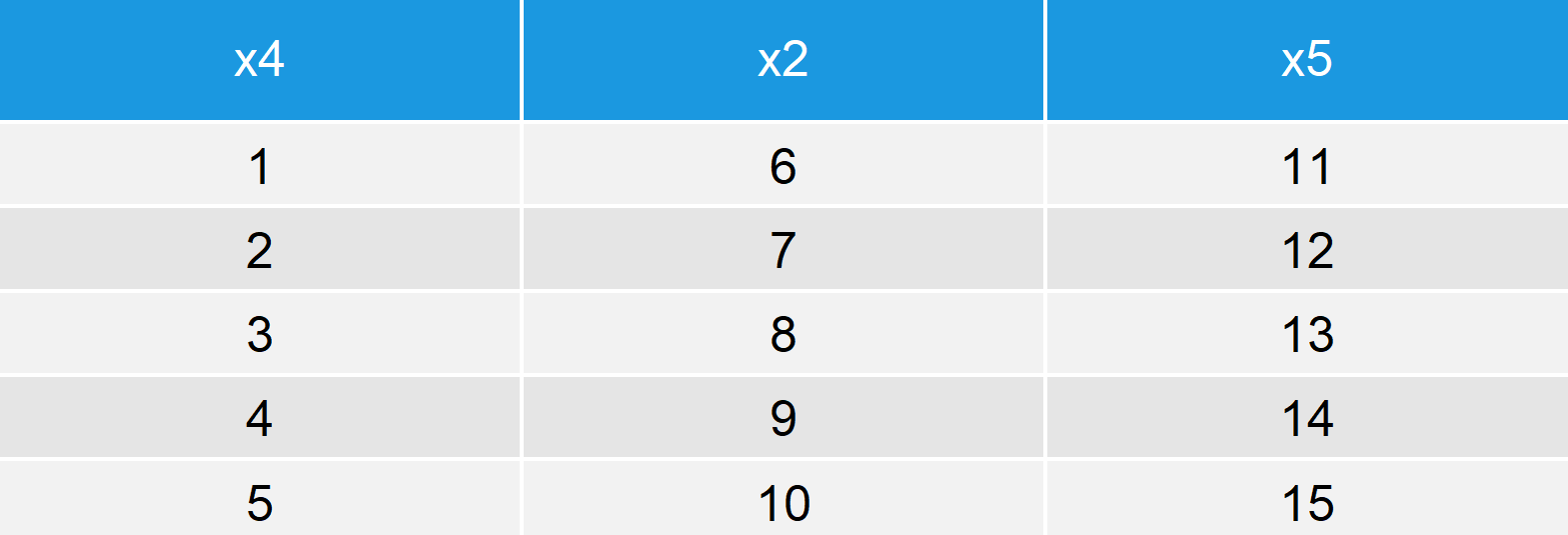# setNames vs. setnames in R (+ Examples) | stats & data.table Package

In this R tutorial, I’ll show you how to apply the setNames function of the R package stats and the setnames function of the R package data.table. The two functions are designed for slightly different situations, which sometimes leads to confusions. That’s why I’m explaining both functions in the same tutorial.

Basic R Syntax of setNames [stats Package]:

`setNames(x, c("name1", "name2", "name3"))`

setNames() sets the names of a data object and returns the object.

Basic R Syntax of setnames [data.table Package]:

`setnames(data, "name_old", "name_new")`

setnames() changes the names of a data.frame or data.table by reference.

In the following R programming tutorial, I’ll show an example for each of the functions.

Let’s start right away…

## Example 1: setNames R Function [stats Package]

In the first example, I’ll illustrate the usage of the setNames R Function of the stats package. Let’s first create an example vector:

```x <- 1:5                                    # Create example vector
x                                           # Print example vector to RStudio console
# 1 2 3 4 5```

Our example vector consists of the numbers 1 to 5. Now let’s assign a name to each of these numbers via setNames():

```x_names <- setNames(x, letters[1:5])        # Apply setNames function
x_names                                     # Print updated vector to RStudio console
# a b c d e
# 1 2 3 4 5```

I have released a video on the Statistics Globe YouTube channel, which explains the R programming code of Example 1 in some more detail. You can check out the video here:

Please accept YouTube cookies to play this video. By accepting you will be accessing content from YouTube, a service provided by an external third party.If you accept this notice, your choice will be saved and the page will refresh.

Easy! So what is the difference compared to the setnames function of the data.table package?

## Example 2: setnames R Function [data.table Package]

These are the main differences between setNames [stats Package] and setnames [data.table Package]:

• setnames of the data.table package has a lower case n instead of an upper case N.
• setnames can only be applied to data.table and data.frame objects.
• setnames changes names by reference.

Let’s do an example…

First, we need to install and load the data.table package. Note that we did not have to do this in Example 1, since the stats package is automatically loaded with base R.

```install.packages("data.table")              # Install data.table package

Now, let’s create an example data.frame for the application of setnames:

```data <- data.frame(x1 = 1:5,                # Create example data.frame
x2 = 6:10,
x3 = 11:15)
data                                        # Print example data.frame to RStudio console```Table 1: Example data.frame Before Application of setnames().

Our example data consists of three columns with the column names x1, x2, and x3.

Now, we can use the setnames R function to rename certain column names:

```setnames(data,                              # Apply setnames function
c("x1", "x3"),
c("x4", "x5"))
data                                        # Print updated example data.frame```Table 2: Example data.frame After Application of setnames().

The colnames x1 and x3 were replaced by x4 and x5.

Check out the following video in case you need further information on the setnames function of the data.table package:

Please accept YouTube cookies to play this video. By accepting you will be accessing content from YouTube, a service provided by an external third party.If you accept this notice, your choice will be saved and the page will refresh.

## Video: Related Functions

As you have seen, setNames and setnames can and should be applied in different data situations. However, there are many other functions that could also be used instead of setNames and setnames. In the following video, I’m showing you how to rename column names in R with the colnames function – one of the alternatives for setnames:

Please accept YouTube cookies to play this video. By accepting you will be accessing content from YouTube, a service provided by an external third party.If you accept this notice, your choice will be saved and the page will refresh.